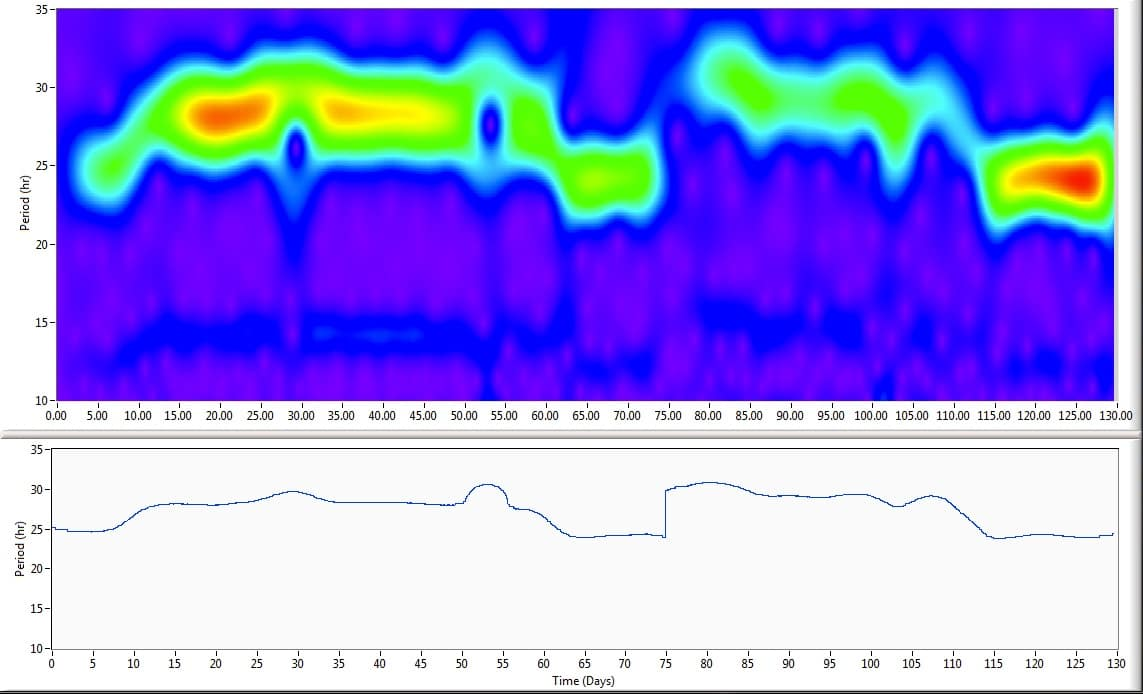## ClockLab Analyses

### Automate Onset/Offset Detection

The red dots show the results of the automated onset-time calculation. The calculations are 95% correct for most data. In this example, one of the times need to be edited (line 45), which is done simply by shift-clicking on the appropriate point within the line for that day. The calculation can also be adjusted for different data sets using the On and Off controls to the bottom left. The underlying actogram is automatically dimmed to increase the visibility of the dots. (The colors of the dots and the activity plot, as well as the onset symbols can all be changed.) With the onset times identified, several useful calculations are now possible, such as fitting the onsets to a line and calculating Tau from the fit line, calculating phase shifts (below).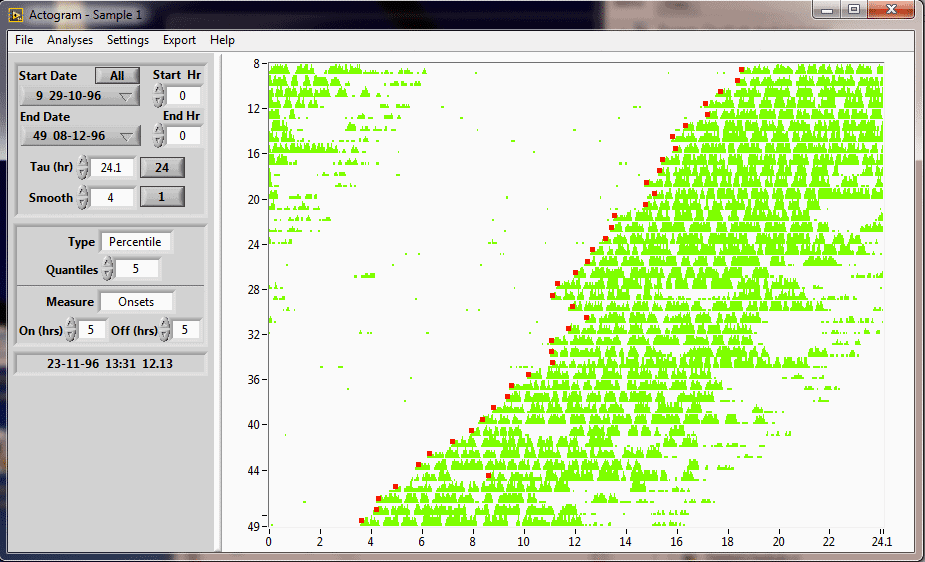### Phase Shifts

With the onset times identified, least-squares fits to the onsets have been calculated for two different time periods. Shift-Clicking on the actogram within the raster for a given day will now display the time delay between the two fitted lines extrapolated to that day. Here, for example, the phase shift of 2.24 hours is being displayed for line 30. The TAU’s for the two fits, here 23.6 and 23.75 hours, are also displayed for the user (not shown). Setting up the fits and displaying the phase difference takes only a few mouse clicks.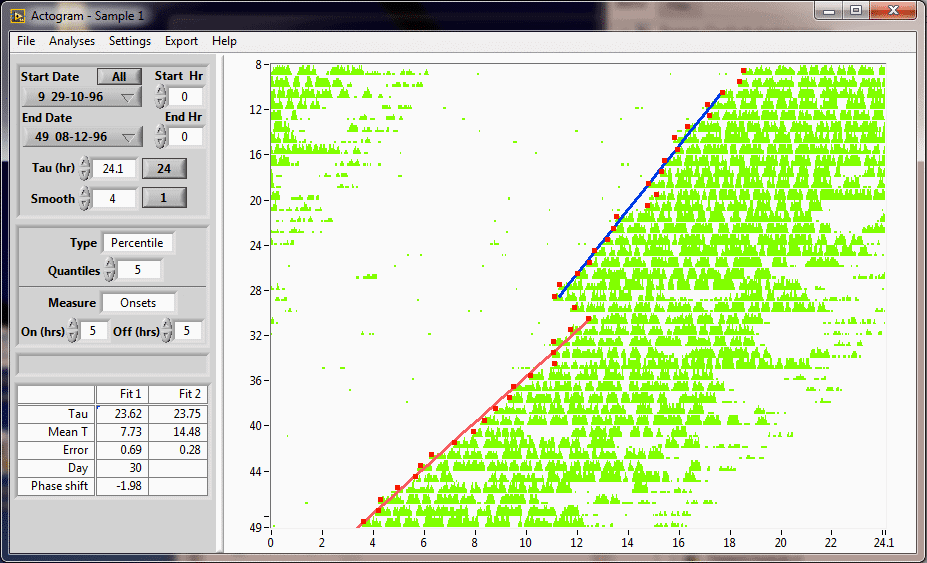### Bout Analysis

The program has identified and displayed the start of activity bouts that exceed a user-defined threshold (here, 5 counts/minute) for a user-defined period (here, 10 minutes). The day, start time, circadian day, circadian start time, number of counts per bout, and bout duration can be exported to a spreadsheet file.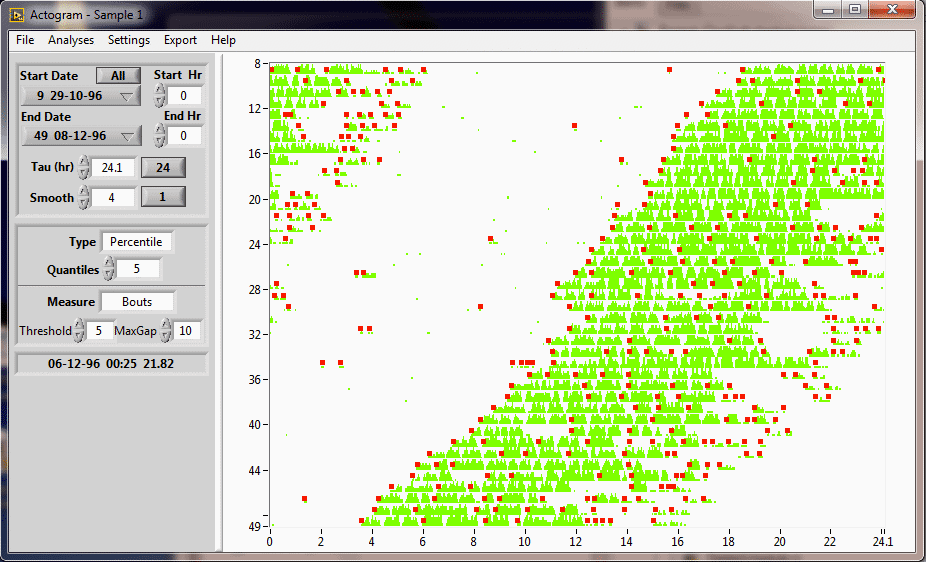### Activity Profile

The Activity Profile shows the average of activity across selected days.

The shading around the activity profile line plot shows the standard deviation of the activity at each point in time.

Cosinor: The red line is a sine-fit to the waveform. Parameters describing the fit are shown in the table to the left, including amplitude, phase, mean and median. In addition, parameters of the Cosinor analysis are listed, including MESOR, the F statistic and an estimate of p-value, which for such a robust rhythm as shown here is arbitrarily close to zero.

NPRCA (Non-Parametric Circadian Rhythm Analysis): NPRCA parameters are listed at the bottom of the analysis table (bottom left), including Relative Amplitude (RA), Interdaily Stability (IS), and Intradaily Variability (IV).

The “Position” and “Counts” indicators at the bottom right show the positions of the two vertical black cursors in the image and the total number of counts for the points between the cursors (alpha counts) and outside the cursors (rho counts).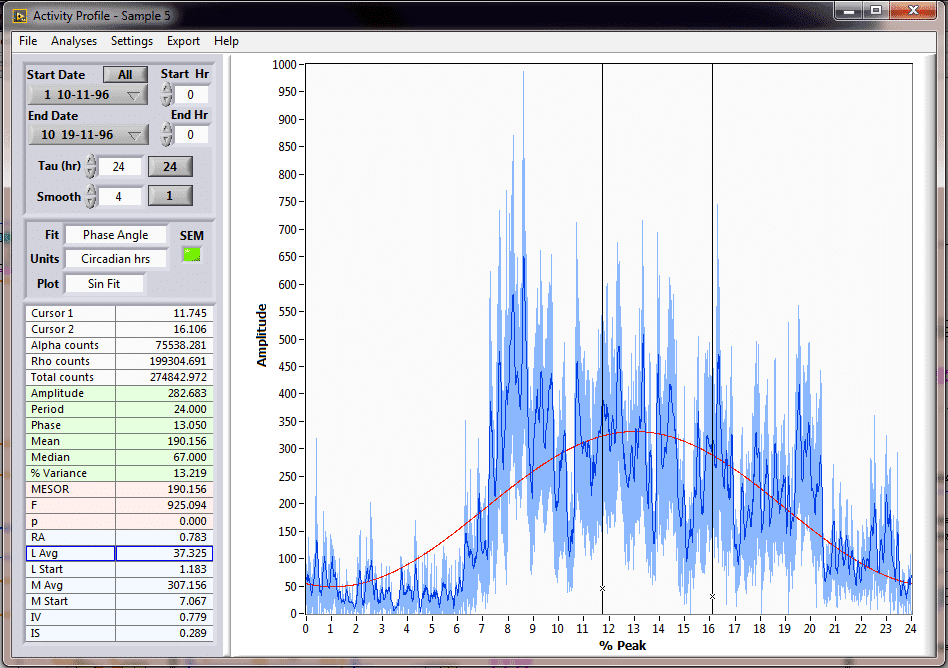### Periodograms

ClockLab can make Chi-square, F or Lomb-Scargle periodograms. The periodogram peak is detected and displayed at the bottom left. The Set Tau button applies this TAU to other open analysis windows, such as the Actogram and Activity Profile, which update automatically with the new Tau. Significance level (green) can be adjusted. The periodogram can be zoomed in to view the peak in detail by clicking and dragging across the image.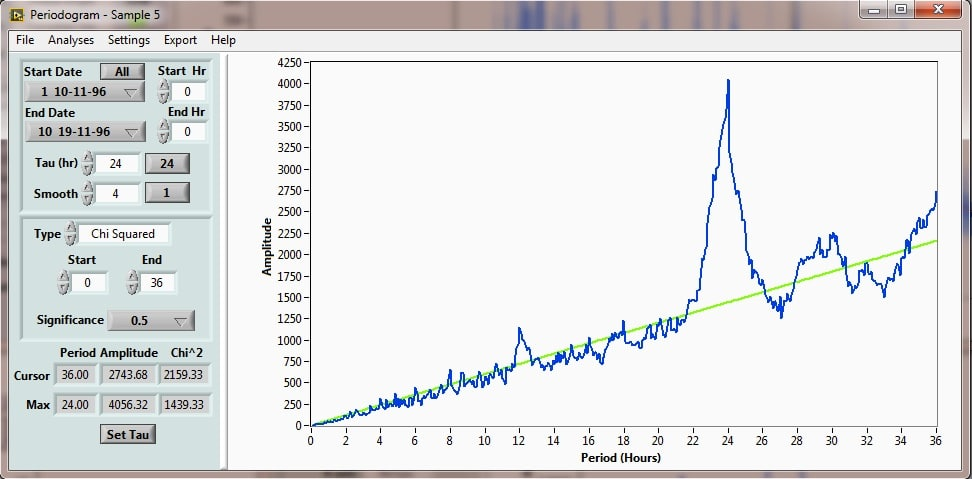### FFT

ClockLab can perform a standard FFT, with or without applying a Blackman-Harris window, and as an amplitude spectrum or power spectrum. The X axis can be set to frequency (cyc/sec) or period (sec/cycle).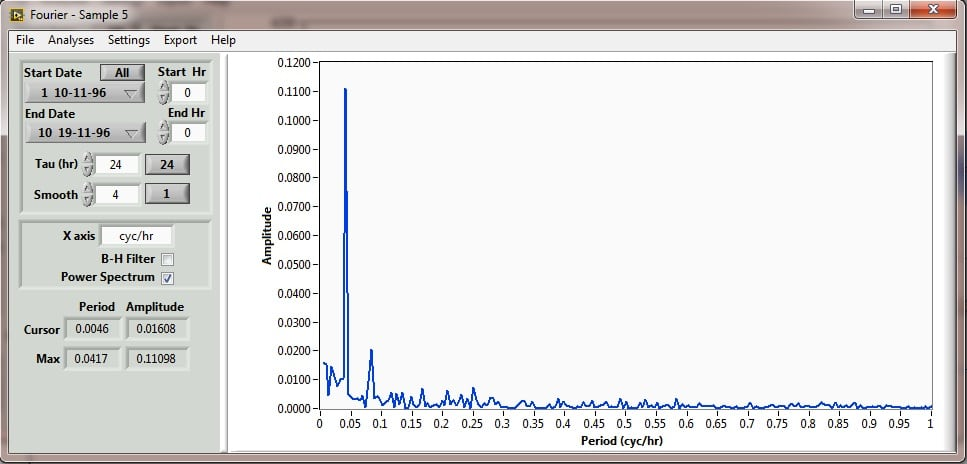### Wavelet Analysis

ClockLab performs a Morlet Continuous Wavelet Transform on the selected portion of the data. ClockLab allows you to adjust the parameters of the wavelet to improve temporal resolution or period resolution.

The 2D  graph (top graph below) color codes the amplitude of the signal at each period as a function of time. The Ridge Plot (bottom) shows the period with the largest amplitude component  at each point in time. This animal free runs in DD at about 28 hours, and runs at 24 hours in LD (days 60-72 and 115-130).